xGRS 80Encyclopedia
GRS 80, or Geodetic Reference System 1980, is a geodetic reference system consisting of a global reference ellipsoid
Reference ellipsoid
In geodesy, a reference ellipsoid is a mathematically-defined surface that approximates the geoid, the truer figure of the Earth, or other planetary body....

and a gravity field model.

## Geodesy

Geodesy
Geodesy
Geodesy , also named geodetics, a branch of earth sciences, is the scientific discipline that deals with the measurement and representation of the Earth, including its gravitational field, in a three-dimensional time-varying space. Geodesists also study geodynamical phenomena such as crustal...

, also called geodetics, is the scientific discipline that deals with the measurement and representation of the earth
Earth
Earth is the third planet from the Sun, and the densest and fifth-largest of the eight planets in the Solar System. It is also the largest of the Solar System's four terrestrial planets...

, its gravitation
Gravitation
Gravitation, or gravity, is a natural phenomenon by which physical bodies attract with a force proportional to their mass. Gravitation is most familiar as the agent that gives weight to objects with mass and causes them to fall to the ground when dropped...

al field and geodynamic phenomena (polar motion
Polar motion
Polar motion of the earth is the movement of Earth's rotational axis across its surface. This is measured with respect to a reference frame in which the solid Earth is fixed...

, earth tide
Tide
Tides are the rise and fall of sea levels caused by the combined effects of the gravitational forces exerted by the moon and the sun and the rotation of the Earth....

s, and crustal motion) in three-dimensional, time-varying space.

The geoid
Geoid
The geoid is that equipotential surface which would coincide exactly with the mean ocean surface of the Earth, if the oceans were in equilibrium, at rest , and extended through the continents . According to C.F...

is essentially the figure of the Earth abstracted from its topographic features. It is an idealized equilibrium surface of sea water, the mean sea level surface in the absence of currents, air pressure variations etc. and continued under the continental masses. The geoid, unlike the ellipsoid, is irregular and too complicated to serve as the computational surface on which to solve geometrical problems like point positioning. The geometrical separation between it and the reference ellipsoid is called the geoidal undulation. It varies globally between ±110 m.

A reference ellipsoid
Reference ellipsoid
In geodesy, a reference ellipsoid is a mathematically-defined surface that approximates the geoid, the truer figure of the Earth, or other planetary body....

, customarily chosen to be the same size (volume) as the geoid, is described by its semi-major axis (equatorial
radius) a and flattening f. The quantity f = (ab)/a, where b is the semi-minor axis (polar radius), is a purely geometrical one. The mechanical ellipticity of the earth (dynamical flattening, symbol J2) is determined to high precision by observation of satellite orbit perturbations. Its relationship with the geometric flattening is indirect. The relationship depends on the internal density distribution, or, in simplest terms, the degree of central concentration of mass.

The 1980 Geodetic Reference System (GRS80) posited a 6,378,137 m semi-major axis and a 1:298.257 flattening. This system was adopted at the XVII General Assembly of the International Union of Geodesy and Geophysics (IUGG). It is essentially the basis for geodetic positioning by the Global Positioning System and is thus also in extremely widespread use outside the geodetic community.

The numerous other systems which have been used by diverse countries for their maps and charts are gradually dropping out of use as more and more countries move to global, geocentric reference systems using the GRS80 reference ellipsoid.

## Defining features of GRS 80

The reference ellipsoid is usually defined by its semi-major axis
Semi-major axis
The major axis of an ellipse is its longest diameter, a line that runs through the centre and both foci, its ends being at the widest points of the shape...

(equatorial
radius)and either its semi-minor axis
Semi-minor axis
In geometry, the semi-minor axis is a line segment associated with most conic sections . One end of the segment is the center of the conic section, and it is at right angles with the semi-major axis...

(polar radius)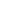, aspect ratio
Aspect ratio
The aspect ratio of a shape is the ratio of its longer dimension to its shorter dimension. It may be applied to two characteristic dimensions of a three-dimensional shape, such as the ratio of the longest and shortest axis, or for symmetrical objects that are described by just two measurements,...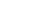or flattening
Flattening
The flattening, ellipticity, or oblateness of an oblate spheroid is a measure of the "squashing" of the spheroid's pole, towards its equator...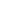, but GRS80 is an exception.

Defining geometrical constants :
Semi-major axis = Equatorial Radius6,378,137.00000 m;

Derived geometrical constants :
Flattening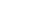0.003352810681225;

Reciprocal of flattening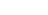298.257222101;

Semi-minor axis = Polar Radius6,356,752.31414 m;

Aspect ratio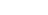0.996647189318816;

Mean radius as defined by the International Union of Geodesy and Geophysics
International Union of Geodesy and Geophysics
The International Union of Geodesy and Geophysics is a non-governmental organisation dedicated to the scientific study of the Earth using geophysical and geodesic techniques. The IUGG was established in 1919. Some areas within its scope are environmental preservation, reduction of the effects of...

(IUGG): R1= (2a+b)/3 = 6,371,008.7714 m;

Authalic mean radius= 6,371,007.1810 m;

Radius of a sphere of the same volume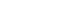6,371,000.7900 m;

Linear eccentricity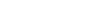521,854.0097 m;

Eccentricity of elliptical section through poles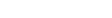0.0818191910435;

Polar radius of curvature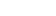6,399,593.6259 m;

Equatorial radius of curvature for a meridian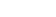6,335,439.3271 m;

Meridian quadrant = 10,001,965.7293 m;

Defining physical
Nature
Nature, in the broadest sense, is equivalent to the natural world, physical world, or material world. "Nature" refers to the phenomena of the physical world, and also to life in general...

constants :
Geocentric gravitational constant, including mass of the atmosphere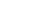3986005·108 m3/s2;

Dynamical form factor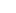= 108263· 10-8;

Angular velocity of rotation= 7292115·10-11 s-1;

For a complete definition, four independent constants are required. GRS80 chooses as these,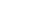,and, making the geometrical constanta derived quantity. The formula is iterative; plugging in the defined constants gives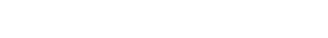where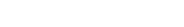exactly.
Replace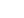with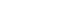and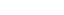with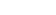and iterate to get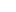; a rounded value comes out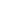0.006694380022903415749574948586289306212443890

from which a rounded value ofcalculates to be the reciprocal of
298.2572221008827112431628366

The GRS80 reference system is used by the Global Positioning System
Global Positioning System
The Global Positioning System is a space-based global navigation satellite system that provides location and time information in all weather, anywhere on or near the Earth, where there is an unobstructed line of sight to four or more GPS satellites...

, in a realization called WGS 84 (World Geodetic System 1984). (The WGS84 ellipsoid is very slightly different, with 1/f = 298.257223563 exactly).